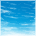# Computing Power dissipation (current consumption) of microcontroller MPC5643L according to the datasheet???

cancel
Showing results for
Did you mean:
SOLVED

## Computing Power dissipation (current consumption) of microcontroller MPC5643L according to the datasheet???

537 ViewsContributor I

Hello to all,

Could someone help me with the Current consumption computation of the micro controller MPC5643L ?

my guess is something like : TotalPowerConsumption = RunModePower + Sleep × deepSleepModePower and it should be some integral shape equation since it won't be a linear and dependent on our time period. I don't know that how can I compute this current with regards to the data sheet.

Regards

Ras

1 Solution
217 ViewsNXP TechSupport

Typical purposes of current consumption computation are to scale power supply or thermal management.

1.

It doesn't matter if the device is sleeping most time or not. Anyway, power supply must be able to provide maximum current consumed by the device.

HV supply should scaled at max(IDD_LV_FULL + IDD_LV_PLL + IDD_HV_xx) current.

80 mA + 2.50 mA*fCPU[MHz] + 10ma + 3ma + 3.5ma + 4ma + 10ma = 410.5ma @ 3.3V, 150'C, 120MHz

Recommended supply current ~450ma.

2.

Thermal management. Use typical current  consumption characteristics.

If LV internal ballast transistor is used:

P_tot = typ (IDD_LV_FULL + IDD_LV_PLL + IDD_HV_xx) * 3.3V

P_tot = (41 ma + 2.3 mA*fCPU[MHz] + 10ma + 3ma + 3.5ma + 4ma + 10ma)*3.3V = 1.15W @ 150'C, 120MHz

If LV external ballast transistor is used:

P_tot = typ (IDD_LV_FULL + IDD_LV_PLL)*1.32V + IDD_HV_xx * 3.3V

P_tot = (41 ma + 2.3 mA*fCPU[MHz])*1.32V + (10ma + 3ma + 3.5ma + 4ma + 10ma)*3.3 = 0.52W @ 150'C, 120MHz

3.

Power consumption estimation in HALT mode.

P_HALT = typ (IDD_LV_HALT + IDD_HV_xx) * 3.3V

P_HALT = (115ma + 30.5ma) * 3.3V = 0.48W @ 150'C, 120MHz

This estimation provides some margin since IDD_HV_xx consumption in sleep modes should be less than in full run mode.

4.

If you want to estimate average consumption in mixed sleep/run mode:

P_average = P_tot * duty + P_HALT*(1-duty)

Note. All estimations are made at Tj=150'C.

Have a great day,
Paul

-----------------------------------------------------------------------------------------------------------------------
-----------------------------------------------------------------------------------------------------------------------

2 Replies
217 ViewsContributor I

Hey Paul,

that's exactly what I needed. Thanks a lot.

Cheers

Ras

218 ViewsNXP TechSupport

Typical purposes of current consumption computation are to scale power supply or thermal management.

1.

It doesn't matter if the device is sleeping most time or not. Anyway, power supply must be able to provide maximum current consumed by the device.

HV supply should scaled at max(IDD_LV_FULL + IDD_LV_PLL + IDD_HV_xx) current.

80 mA + 2.50 mA*fCPU[MHz] + 10ma + 3ma + 3.5ma + 4ma + 10ma = 410.5ma @ 3.3V, 150'C, 120MHz

Recommended supply current ~450ma.

2.

Thermal management. Use typical current  consumption characteristics.

If LV internal ballast transistor is used:

P_tot = typ (IDD_LV_FULL + IDD_LV_PLL + IDD_HV_xx) * 3.3V

P_tot = (41 ma + 2.3 mA*fCPU[MHz] + 10ma + 3ma + 3.5ma + 4ma + 10ma)*3.3V = 1.15W @ 150'C, 120MHz

If LV external ballast transistor is used:

P_tot = typ (IDD_LV_FULL + IDD_LV_PLL)*1.32V + IDD_HV_xx * 3.3V

P_tot = (41 ma + 2.3 mA*fCPU[MHz])*1.32V + (10ma + 3ma + 3.5ma + 4ma + 10ma)*3.3 = 0.52W @ 150'C, 120MHz

3.

Power consumption estimation in HALT mode.

P_HALT = typ (IDD_LV_HALT + IDD_HV_xx) * 3.3V

P_HALT = (115ma + 30.5ma) * 3.3V = 0.48W @ 150'C, 120MHz

This estimation provides some margin since IDD_HV_xx consumption in sleep modes should be less than in full run mode.

4.

If you want to estimate average consumption in mixed sleep/run mode:

P_average = P_tot * duty + P_HALT*(1-duty)

Note. All estimations are made at Tj=150'C.

Have a great day,
Paul

-----------------------------------------------------------------------------------------------------------------------